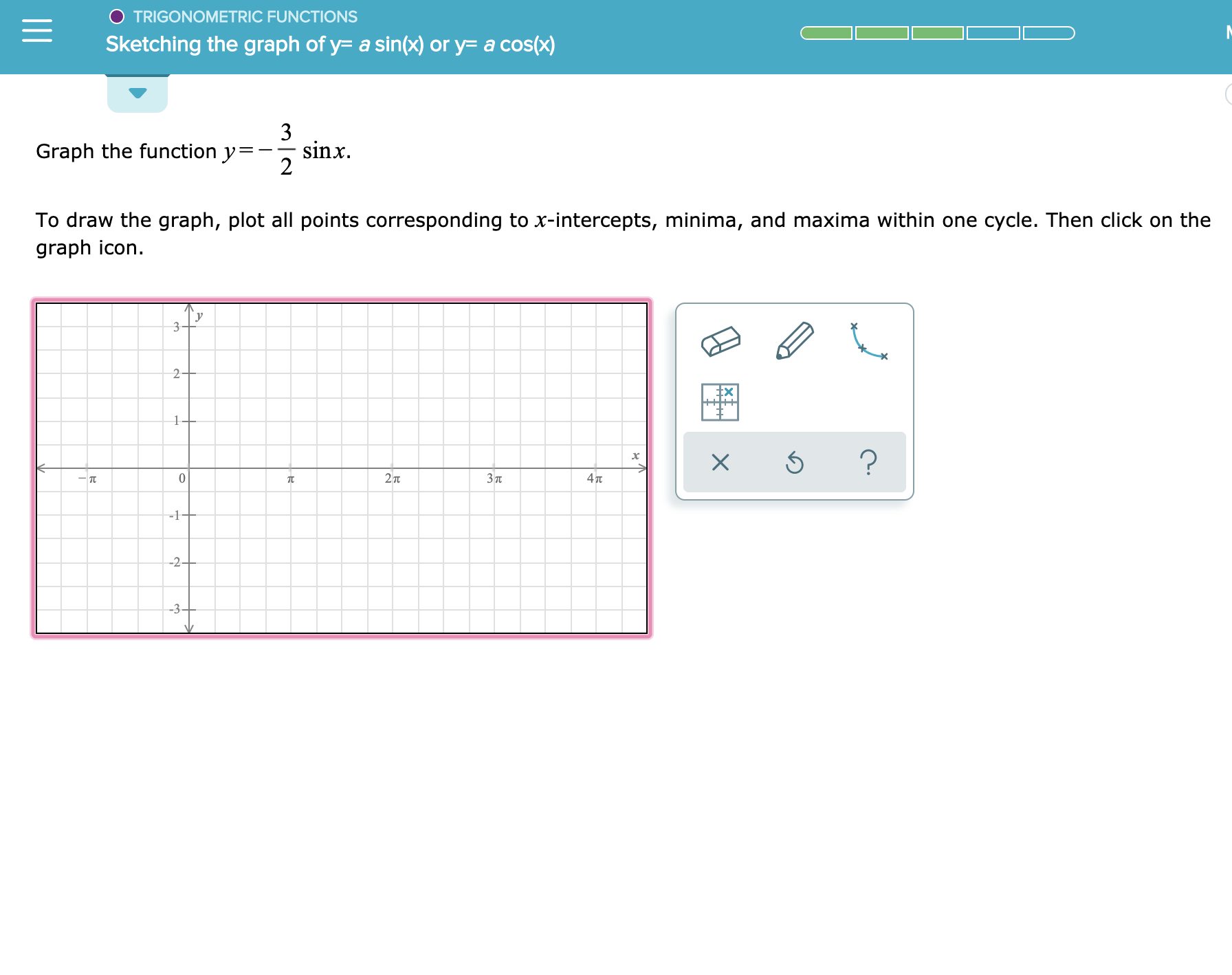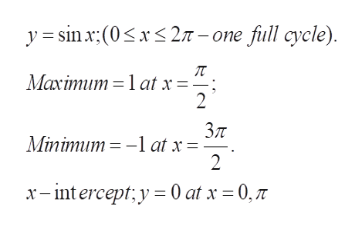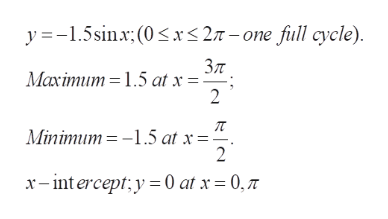# TRIGONOMETRIC FUNCTIONSSketching the graph of y a sin(x) or y= a cos(x)3sinxGraph the function y=2To draw the graph, plot all points corresponding to x-intercepts, minima, and maxima within one cycle. Then click on thegraph icon32Ex?027TЗп4TT-1--2+-3-

Question
1 views

See attachmenthelp_outlineImage TranscriptioncloseTRIGONOMETRIC FUNCTIONS Sketching the graph of y a sin(x) or y= a cos(x) 3 sinx Graph the function y= 2 To draw the graph, plot all points corresponding to x-intercepts, minima, and maxima within one cycle. Then click on the graph icon 3 2 Ex ? 0 27T Зп 4TT -1- -2+ -3- fullscreen
check_circle

Step 1

to study the function y= -1.5 sin(x), for one cycle and graph the same

Step 2

First consider the basic function y=sin x (the given function is just a rescaling of y=sinx).help_outlineImage Transcriptionclosey sin:(0x27-one full cycle) Maximum1at x= 2 Зл at x = 2 Minimum x-intercept y0 at x 0,7 fullscreen
Step 3

Note the given function is (-1.5) sinx; so the minimum and the maximum...help_outlineImage Transcriptionclosey 1.5sn (0< 27-one full cycle) Зл Maximum1.5 at x= 2 Minimum1.5 at x= 2 x-int ercepty = 0 at x= 0, 7 fullscreen

### Want to see the full answer?

See Solution

#### Want to see this answer and more?

Solutions are written by subject experts who are available 24/7. Questions are typically answered within 1 hour.*

See Solution
*Response times may vary by subject and question.
Tagged in

### Functions